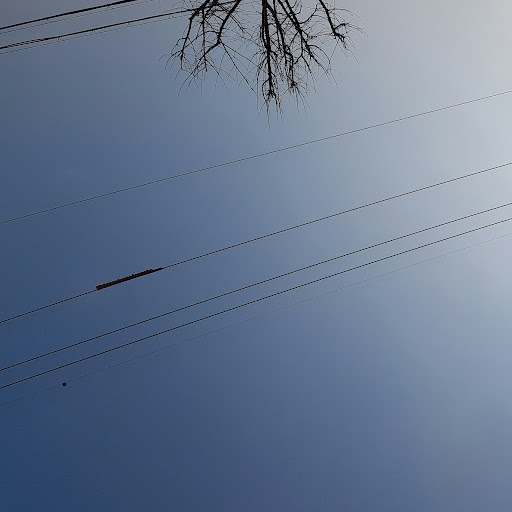# Simple recursive function not giving expected output

Sorry if I did this wrong, i'm really new with C and haven't used stack overflow before. I'm trying to trace this simple recursive function by hand but am getting a different answer from the compiled code.

My thought process was

print 2 | n = 2-1 = 1 | 1 >= 0 | countdown(1)

print 1 | n = 1-1 = 0 | 0 >= 0| countdown(0)

print 0 | n = 0-1 = -1 | -1 is not >= 0|

print -1| END

``````void countdown(int n)
{
printf ("n = %d\t", n);
n--;
if (n >= 0)
{
countdown(n);
}
printf ("n = %d\t", n);
}

int main ( )
{
countdown(2);
return 0;
}
``````

I expected to get: n = 2 n = 1 n = 0 n = -1

but the compiled code gives me: n = 2 n = 1 n = 0 n = -1 n = 0 n = 1

I'm not quite sure where the additional 0 and 1 come from after -1On

Your code has no problem. Just remove 2nd `printf` code.

``````void countdown(int n)
{
printf("n = %d\t", n);
n--;
if (n >= 0)
countdown(n);
}

int main()
{
countdown(2);
return 0;
}
``````

The result is:

``````n = 2 n = 1 n = 0
``````

This is the callstack when I captured at `2nd printf`.

``````StudyCpp.exe!countdown(int n) line 16   C++  // It is 2nd printf of countdown(0). Now, n is -1.
StudyCpp.exe!countdown(int n) line 14   C++  // It called countdown(0)
StudyCpp.exe!countdown(int n) line 14   C++  // It called countdown(1)
StudyCpp.exe!main() line 21 C++   // It called countdown(2)
``````

If you progress debug one more, You can see the callstack as below:

``````StudyCpp.exe!countdown(int n) line 16   C++  // It is 2nd printf of countdown(1) after executed countdown(0).
StudyCpp.exe!countdown(int n) line 14   C++  // It called countdown(1)
StudyCpp.exe!main() line 21 C++   // It called countdown(2)
``````

And, If you progress debug one more, You can see the callstack as below:

``````StudyCpp.exe!countdown(int n) line 16   C++  // It is 2nd printf of countdown(2) after executed countdown(1).
StudyCpp.exe!main() line 21 C++   // It called countdown(2)
``````

And, Finally, Program will be exited.On

Your code does the following (omitting the if):

``````countdown(n):
print(n)
countdown(n-1)
print(n-1)
``````

For n=2:

``````countdown(2):
print(2)
countdown(1)
print(1)

-> next recursion step
print(2)
print(1)
countdown(0)
print(0)
print(1)

-> next recursion step
print(2)
print(1)
print(0)
print(-1)
print(0)
print(1)
``````On

Here you are calling countdown() recursively and is called thrice.
Actually each recursive call will push countdown() into the stack.

``````SF1(Bottom of stack) ---> SF2 ---> SF3 (Top of stack).
``````

Now the frame in the top will be executed.
During the return from the function, the particular stack frame will be popped out.
Now Stack frame pointer points to SF2 and then SF1.

Considering your program, below is the flow.
Push Operation:
SF1 will be pushed onto the stack first

``````n = 2 printed.
Again n updated to 1
``````

SF2 pushed:

``````n = 1 got printed.
Again n updated to 0
``````

SF3 pushed:

``````n = 0 got printed.
Again n updated to -1.
But n <= 0, So if check fails.
``````

Pop operation:
Now SF3 got popped out from stack first.

``````n = -1 printed
``````

Then SF2 popped

``````prints n = 0.
``````

Finally SF1

``````n = 1 printed
``````